American Journal of Applied Mathematics
Volume 4, Issue 2, April 2016, Pages: 75-79

Hypographs of Upper Semi-continuous Maps and Continuous Maps on a Bounded Open Interval

Department of Mathematics and Statistics, Hanshan Normal University, Chaozhou, Guangdong, China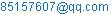Nada Wu. Hypographs of Upper Semi-continuous Maps and Continuous Maps on a Bounded Open Interval. American Journal of Applied Mathematics. Vol. 4, No. 2, 2016, pp. 75-79. doi: 10.11648/j.ajam.20160402.12

Received: February 3, 2016; Accepted: March 21, 2016; Published: March 25, 2016

Abstract: For any bounded open interval X in the Euclidean space E1, let ↓USC(X) and ↓C(X) be the families of all hypographs of upper semi-continuous maps and continuous maps from X to I=[0,1], respectively. They are endowed with the topology induced by the Hausdorff metric of the metric space Y×IY is the closure of X. It was proved in other two papers respectively that ↓USC(X) and ↓C(X) are homeomorphic to s and c0 respectively, where s=(-1,1) and c0={(xn)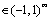: limn→∞xn=0}. However the topological structure of the pair (↓USC(X), ↓C(X)) was not clear. In the present paper, it is proved in the strongly universal method that the pair of spaces (↓USC(X), ↓C(X)) is pair homeomorphic to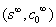which is not homeomorphic to (s, c0). Hence this paper figures out the topological structure of the pair (↓USC(X), ↓C(X)).

Keywords: Hypograph, Upper Semi-continuous Maps, Continuous Maps, Bounded Open Interval, Hausdorff Metric, The Property of Strongly Universal

Contents

1. Introduction

For a Tychonoff space X and a subspace L of the real line R with the usual topology, let C(X, L) denote the set of all continuous maps from X to L. C(X, L) can be endowed with different topologies which are interesting for topologists. There are many research results in this field. In 1966 and 1991, two classical results were proved respectively which are listed below.

Theorem 1 [Anderson-Kadec Theorem] . Cu(X, L) is homeomorphic to (≈) the Hilbert space l2≈s=(-1,1)  if X is an infinite compactum (a compactum means a compact metric space) and L=I=[0,1] or L=R, where Cu(X, L) is C(X, L) endowed with the uniformly convergence topology.

Theorem 2 . Cp(X, L)≈c0 if X is a countable non-discrete metric space and L=R or L=I, where Cp(X, L) denotes C(X, L) endowed with the pointwise convergence topology, and c0={(xn): limn→∞xn=0}.

In  to , C(X, I) was endowed with another topology. To introduce this topology, it is necessary to recall the knowledge about hyperspace. For a metric space (M, d), the hyperspace Cld(M) is the set consisting of all non-empty closed subsets of M endowed with Vietoris topology. Hausdorff distance dH defined as follows:

dH(A, B)=Inf{ε: Bd (A, ε)B and Bd(B, ε)A}  (1)

for any A, BCld(M). It is well-known that if M is compact, then dH is a metric and deduces Vietoris topology on Cld (M).

Let X be a space. A (single-valued) function f: X → R is called upper semi-continuous if f−1(−∞, t) is open in X for every tR. For a Tychonoff space X and LR, let USC(X, L) denote the family of all upper semi-continuous maps from X to L. For convenience, USC(X, I) and C(X, I) are abbreviated as USC(X) and C(X) respectively.

For every f USC(X), let ↓f be the region below of f, that is, ↓f = {(x, λ)X×I: λ ≤ f (x) } then ↓f Cld (X×I).

Hence↓USC(X)={↓f: fUSC(X)} and ↓C(X)= {↓f: fC(X)} can be topologized as subspaces of the hyperspace Cld(X×I). ↓C(X) can be considered as C(X) endowed with another topology which is different with the two former topologies ([4, Corollary 1]).

For two pairs of spaces (X1, Y1) and (X2, Y2) with Y1X1 and Y2X2, the symbol (X1, Y1)≈(X2, Y2) means that there exists a homeomorphism h: X1 →X2 such that h(Y1)=Y2. For a metric space X, we use X0 and clX(·) to denote the set of all isolated points of X and the closure-operator in X, respectively.

In 2005, it was proved that ↓USC(X)≈Q (Q = [-1, 1]is the Hilbert cube) when X is a compactum .

In 2006, there was the following result.

Theorem 3 . For a Tychonoff space X, the following

conditions are equivalent:

(a) X is a compactum and clX(X0)≠X;

(b) ↓C(X) ≈ c0;

(c) (↓USC(X), ↓C(X)) ≈ (Q, c0).

In 2009, the following theorem was proved which indicated that the topological structure of (↓USC(X), ↓C(X)) was figured out for every compactum X.

Theorem 4 . Let X be a compact metric space, |X| denotes the cardinal number of X, and Σ={(xn)Q: sup{xn: nN+} < 1} be a subspace of Q, thenIf X is a non-compact space, ↓USC(X) and ↓C(X) can be endowed with Fell topology. From 2014 to 2016,Yang made a series of research in this area, see [9-11].

If X is a non-compact and topological complete metric space whose completion is compact, then ↓USC(X) and ↓C(X) can be embed in ↓USC(Y) as subspaces [12,13], where Y is the completion of X. In fact, define a map ↓e: ↓USC(X)→↓USC(Y) as following: for any f USC(X),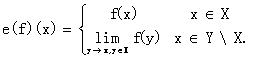(2)

It had been proved in  that ↓e is an isometric imbedding.

In 2008, there was the following result about ↓USC(X).

Theorem 5 . ↓USC(X) ≈ l2 ≈s if (X, ρ) is a non-compact and topological complete metric space whose completion is compact.

However, at that time, we didn’t know the topological structure of ↓C(X) for every X satisfying the condition in Theorem 5. In 2013, we considered the case of clX(X0)≠X, and got the following result.

Theorem 6 . If X is a noncompact, locally compact, totally bounded, separable metric space, then ↓C(X, I) ≈ c0 if and only if clX(X0)≠X.

We failed to prove that (↓USC(X,I),↓C(X,I))≈(s,c0) for every noncompact, locally compact, totally bounded, separable metric space X with clX(X0)≠X. In this paper, we take a particular case, that is, consider X as a bounded open interval in the Euclidean space E1 and we prove the following main result.

Theorem 7. For any bounded open interval X in the Euclidean space E1, (↓USC(X), ↓C(X))≈.

Remark 1. In fact (Q, s) ≈ (Q, s) and (Q, c0) ≈ (Q, c0), however, (s, c0) is not pair homemomorphic to (s, c0) . Hence the above theorem indicates that (USC(X), C(X)) is not homeomorphic to (s, c0).

2. Preliminaries

All spaces under discussion are assumed to be separable metrizable spaces. All definitions on this section can be found in  or .

Definition 1 A space X is called an absolute retract, abbreviated AR, provided that for every space Y containing X as a closed subspace, X is a retract of Y, that is, there exists a continuous map r: Y → X such that r|X = idX.

Definition 2 A closed subset A of a space X is said to be a Z-set of X if the identity idX can be approximated by continuous maps from X to X \ A. A Zσ-set in a space is a countable union of Z-sets in the space. A space is called a Zσ−space if it is a Zσ−set of itself. We use Z(X) and Zσ(X) to denote the family of all Z-sets and the family of all Zσ-sets in X, respectively. A Z-embedding is an embedding with a Z-set image.

Definition 3 A subset A of a space Y is called homotopy dense in Y if there exists a homotopy h: Y × I → Y such that h0 = idY and ht(Y)A for every t > 0.

Definition 4 Let M0 and M1 denote the class of compacta and the class of topological complete spaces, respectively. A space is called an absolute Fσ-space if it is an Fσ-set in any space which contains it as a subspace. A space is called an absolute Fσδ-space if it is an Fσδ-set in any space which contains it as a subspace. Let M2 denote the class of all absolute Fσδ-spaces. Let (M0, M1, M2) denote the class of all triples of spaces (M, B, A) such that MBA, MM0, BM1 and AM2.

Definition 5 Let Fσ denote the class of all absolute Fσ-spaces. Let (X, d) be a copy of Hilbert cube Q and the pair of spaces (X, Y)(M0, M2)(or res. (M0, Fσ)). We say that (X, Y) is strongly (M0, M2) -universal (or res. strongly (M0, Fσ) universal)provided for each(M, B)(M0, M2) (or res. (M0, Fσ)), each continuous map f: M → X, each closed subset K of M such that f|K: K→X is a Z-embedding and each ε > 0, there is a Z-embedding g: M → X such that g|K = f|K, g−1(Y)\K = B\K and d(g(m), f(m)) < ε for each mM.

Remark 2. (Q, c0) is strongly (M0, M2) –universal  and (Q,Σ) is strongly (M0, Fσ) universal .

Definition 6 Let (X, d) be a copy of Hilbert cube Q and the triple of spaces (X, Y, Z)(M0, M1, M2). We say that (X, Y, Z) is strongly (M0, M1, M2) -universal provided for each

(M, B, A)(M0, M1, M2), each continuous map f: M → X, each closed subset K of M such that f|K: K→X is a Z-embedding and each ε > 0, there is a Z-embedding

g: M → X such that g|K = f|K, g−1(Y) \ K = B \ K, g−1(Z) \ K = A \ K and d(g(m), f(m)) < ε for each m M.

Let Ω2 be the absorbing set in R for the class M2, constructed in .

The following lemma is a statement in [18, page 274].

Lemma 1. Let (RC, R, D) be a triple of spaces with D≈Ω2, then (RC, R, D)≈(RC, R, Ω2) (triple homeomorphism whose definition is similar as pair homeomorphism) if and only if (RC, R, D) is strongly (M0, M1, M2) –universal, RC= [−∞, +∞].

Corollary 1. Let (A, B, C) be a triple of spaces with (A, B) ≈ (Q, s) and C ≈ c0, then (B,C) ≈ (s, c0) if (A, B, C) is strongly (M0, M1, M2) -universal.

Proof. Since (A, B) ≈ (Q, s) ≈ (RC, R), there exists a homeomorphism h: A → RCsuch that h[B] = R. Put D = h[C], then (A, B, C) ≈ (RC, R, D) and D ≈ c0. If (A, B, C) is strongly (M0, M1, M2)-universal then (RC, R, D) is also strongly (M0, M1, M2)-universal. Note that Ω2 ≈ c017, (RC,R, D) ≈ (RC, R, Ω2) by lemma 1. Since (R, Ω2) ≈ (s, c0) , (B, C) ≈ (R, D) ≈ (R, Ω2) ≈ (s, c0).

3. Proof of the Main Result

In this section, X is always assumed for an bounded open interval in E1, and Y be the closure of X which is compact. We can define a metric d on the product space Y×I as following,

d((x1,t1),(x2,t2))=Max{|x1-x2|,|t1-t2|}

for any (x1, t1),(x2, t2)Y×I.

Let dH be the Hausdorff metric on Cld(Y×I).

3.1. Some Lemmas for Proof of Theorem 3

Lemma 2 [13, Corollary 3]. There exists homotopy

H: ↓USC(Y) × I →↓USC(Y) such that

H0 = id↓USC(Y), and Ht(↓USC(Y))↓C(Y) for each t > 0, and dH(H(↓f, t), ↓f)≤t for each fUSC(Y) and each tI.

Lemma 3 [5, Lemma 8]. Let A be a metric space and

a, b: A → I two continuous maps with a(y) < b(y) for each y A. And let M: A × I → I be a map satisfying the following conditions:

(1) for each fixed y0 A, M(y0, t): I → I is increasing,

(2) for every fixed t0 I, M(y, t0): A → I is continuous.

Then s: A→ I defined by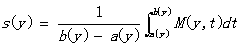is continuous and M(y, a(y)) ≤ s(y) ≤ M(y, b(y)) for every yA.

Lemma 4 [19, lemma 2.9]. (Qu, c1) ≈ (Q, c0), where

Qu =[0,1], c1 = {(xn) Qu: limn→∞ xn = 1}.

Lemma 5 [15, Proposition 5.4.6]. (Q,Q\s) ≈ (Q,Σ).

Lemma 6 [4, Lemma 5]. Let F = EZ↓USC(Y) be closed. If Z is a Z-set in ↓USC(Y) and for every ↓fE there exists aY such that f(a) = 0, then F is a Z-set in ↓USC(Y).

3.2. Proof of Theorem 3

Since (↓USC(Y), ↓USC(X))≈(Q, s) , ↓C(X)≈ c0 , by Corollary 1, it suffices to prove the following lemma.

Lemma 7. The triple of spaces (↓USC(Y), ↓USC(X), ↓C(X)) is strongly (M0, M1, M2) -universal.

Proof. Without loss of generality, assume that X = (−1, 1), Y= [−1, 1]. Let xn = 1/2n, x′n = 1/2n − 1 for every n N+, x0 = 0 and x = −1, then limn→∞ xn = x0 and limn→∞ x′n = x. Let (D, B, A) be a triple of spaces such that (D, B, A)(M0, M1, M2) and K be a closed subset of D. Let Φ: D → USC(Y) be a map such that ↓Φ: D →↓USC(Y) is continuous and ↓Φ| K: K →↓USC(Y) is a Z-embedding. By [7, Lemma 1.1], without loss of generality, we may assume that ↓Φ(K) ∩ ↓Φ(D\K) =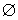. For every ε (0,1), let δ: Y → [0,1) be a map defined by

δ(y) =(1/5)min{ε,d H (↓Φ(y),↓Φ(K))}.

Then δ is continuous and δ(y) = 0 if and only if y K. For every k N+, let

Dk = {y Y: 2−k ≤ δ(y) ≤ 2−k+1}.

Then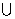kN + Dk= D\K. In what follows, we shall define.

In what follows, we shall define Ψk:Dk → USC(Y) for every k N +, and then use these maps and Φ| K to define a map Ψ:D → USC(X) such that ↓Ψ: D →↓USC(Y) is a Z-embedding, Ψ|K = Φ|K, Ψ −1 (USC(X))\K=B\K, Ψ−1 (C(X)) \K=A\K and d H (↓Ψ(y), ↓Φ(y)) < ε for each yD. This task will be finished in four steps.

It follows from Lemma 3, that there exists a homotopy H: ↓USC(Y) × I →↓USC(Y) such that

H0= id↓USC(Y), Ht(↓USC(Y))↓C(Y) and

dH(Ht(↓f), ↓f) ≤ t for each f USC(Y) and each t (0,1].

For each yD, and tI, let

↓h(y) = H(↓Φ(y),δ(y)),

M 0 (y,t) = sup{h(y)(x): |x − x 0 | < t},and

M(y,t) = sup{h(y)(x): |x − x| < t}.

Then h(y)C(Y) for each yD\K and

↓h| D \K: D\ K →↓C(Y) is continuous.

Moreover, dH(↓h(y),↓Φ(y)) ≤ δ(y) for every yD. It follows from the continuities of δ and H that

M0, M: (D\ K) × I → I satisfies the conditions (1) and (2) in Lemma 3.

Thus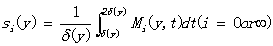is continuous on D\ K and Mi(y,δ(y)) ≤ si(y) ≤ Mi (y,2δ(y)) for every y D \ K.

By Definition 5 and Lemmas 4 and 5, there exist Z-embeddings α: D → Qu and β: D → Q such that

α−1 [c 1] = A and β−1 [Q\Σ] = B. If α(y) = (x1, x2, ···,xn, ···) Q u and β(y) = (z 1, z 2, ···,z n, ···) Q, then the symbols α(y)(n) and β(y)(n) denote x n and z n, respectively. For each n N +, define a map V n: D → I by

Vn (y) = max{|β(y)(i)|: i {1,2,· · ·,n}}

for every yD. It is easy to check that Vn is continuous and lim n→∞ Vn(y) = 1 if and only if yB.

Define continuous maps ϕk: Dk → I by

ϕk (y) = 2 − 2k δ(y). Since y D k, 2−k ≤ δ(y) ≤2−k+1. Hence 0 ≤ ϕ k (y) ≤ 1, ϕ k (y) = 0 if δ(y) = 2−k+1 and ϕk (y) = 1 if δ(y) = 2−k.

Let Yi = {x Y: 2−i−1 ≤ |x − x 0 | ≤ 2−i}, and

Y′i = {x Y: 2−i−1 ≤ |x − x | ≤ 2−i}, then

Y = (1/2, 1]iN + (Y i Y′ i).

Define φi: Y i → I by φi (x) = 2i+1 (2−i − |x − x 0 |) for each iN +. Then φ i (x i) = 0 and φi (x i+1) = 1 for every i ≥ 1.

Define γi: Y′ i → I by γi (x) = 2i+1 (2−i − |x − x |) for each iN+. Then γi (x′i) = 0and γi (x′i+1) = 1 for every i ≥ 1.

Step 2: For every k N+, define a map Ψk on Dk.

For every k N+, define a map Ψk: Dk → USC(Y) as follows:

for every yD k,

if x(1/2, 1]i{1,2,…,2k}(Y i Y′ i),

Ψk (y)(x) = h(y)(x).

Ψ k (y)(x 2k+1) = h(y) (x 2k+1);

Ψ k (y)(x 2k+2) = (1 − ϕ k (y))s 0(y) + ϕ k (y)h(y) (x 2k+2);

Ψ k (y)(x 2k+3) = (1 − ϕ k (y))δ(y) + h(y) (x 2k+3) ϕ k(y);

Ψ k(y)(x 2k+4) = s 0 (y);

Ψ k(y)(x 2k+5) = δ(y);

Ψk(y)(x2k+6) = (1 − ϕ k (y))δ(y)α(y)(1) + ϕ k(y)s0(y);

if m is odd and m ≥ 7, Ψk(y)(x 2k+m) = δ(y);

if m is even and m ≥ 8,

Ψ k (y)(x2k+m) = δ(y)[(1 − ϕk(y))α(y)((m+2)/2− 3)

+ ϕk(y)α(y)(m/2− 3)];

Ψk(y)(x0) = δ(y);

if xY2k+i, i N +,

Ψk(y)(x) = (1− φi(x))Ψk(y)(x2k+i) + φ i(x)Ψk(y)(x2k+i+1).

Ψk(y)(x′2k+1) = h(y) (x′2k+1);

Ψk(y)(x′2k+2) = (1− ϕk(y))s(y) + ϕk(y)h(y)(x′2k+2);

Ψk(y)(x′2k+3) = h(y)(x′2k+3k(y);

Ψk(y)(x′2k+4) = s(y);

Ψk(y)(x′2k+5) = 0;

Ψk(y)(x′2k+6) = (1− ϕk(y))δ(y)V1(y) + ϕk(y)s(y);

if m is odd and m ≥ 7, Ψk(y)(x′2k+m) = 0;

if m is even and m ≥ 8,

Ψk (y)(x′2k+m)= δ(y)[(1 − ϕk(y))V ((m+2)/2−3) (y)

+ ϕk(y)V (m/2 −3) (y)];

Ψ k (y)(x) = δ(y);

if x Y′2k+i, iN+,

Ψk(y)(x)=(1 −γi (x)) Ψk(y) (x′2k+i)

+ γi(x)Ψk(y)(x′2k+i+1).

Step 3: Checking corresponding properties of maps Ψk′s to prepare for defining the map Ψ.

Claim 1: For every yDk, Ψk(y) is well-defined on Y, continuous on Y\{x0, x} and upper semi-continuous at x0 and x. Moreover, lim x→x∞Ψk(y)(x) = δ(y) =Ψk(y)(x) if and only if limn→∞Vn(y)=1 if and only if yB. Therefore, if y Dk, then Ψk(y)USC(X) if and only if yB∩Dk. Ψk(y) is continuous at x0 if and only if limn→∞α(y)(n)=1 if and only if yA∩Dk.

The proof of Claim 1 is trivial.

Claim 2: For each kN+, ↓Ψk:Yk →↓USC(Y) is continuous.

It can be proved like the proof of [4, Proposition 1].

Claim 3: Ψk(y) = Ψk+1(y) for every yDk∩Dk+1.

For every yDk∩D k+1, we have δ(y) =1/2k.

Thus, ϕk(y) = 1 and ϕk+1(y) = 0.

If x(1/2, 1]i{1,2,…,2k}(Y i Y′ i),

Ψk(y)(x) = h(y)(x)=Ψk+1(y)(x).

Ψ k (y)(x 2k+1) = h(y) (x 2k+1)k+1(y)(x 2k+1).

Ψ k (y)(x 2k+2)= h(y) (x 2k+2)= Ψk+1(y)(x 2k+2).

Ψ k (y)(x 2k+3)= h(y) (x 2k+3)= Ψk+1(y)(x 2k+3).

Ψ k(y)(x 2k+4) = s 0 (y) = Ψk+1(y)(x 2k+4).

Ψ k(y)(x 2k+5) = δ(y)= Ψk+1(y)(x 2k+5).

Ψk(y)(x2k+6)= s 0 (y) =Ψk+1(y)(x2k+6).

If m is odd and m ≥ 7,

Ψk(y)(x 2k+m) = δ(y)= Ψk+1(y)(x 2k+m).

If m is even and m ≥ 8,

Ψ k (y)(x2k+m) = δ(y)α(y)(m/2−3) =Ψ k+1 (y)(x2k+m).

Ψk(y)(x0) = δ(y)= Ψk+1(y)(x0).

If xY2k+i = Y2(k+1)+(i-2),iN +,

Ψk(y)(x) = (1− φi(x))Ψk(y)(x2k+i) + φ i(x)Ψk(y)(x2k+i+1)

= (1− φi(x))Ψk+1(y)(x2(k+1)+(i-2))

+ φ i(x)Ψk+1(y)(x2(k+1)+(i-2)+1)

k+1(y)(x).

Ψk(y)(x′2k+1) = h(y) (x′2k+1)= Ψk+1(y)(x′2k+1);

Ψk(y)(x′2k+2) = h(y)(x′2k+2) =Ψk+1(y)(x′2k+2).

Ψk(y)(x′2k+3) = h(y)(x′2k+3) =Ψk+1(y)(x′2k+3).

Ψk(y)(x′2k+4) = s(y) =Ψk+1(y)(x′2k+4).

Ψk(y)(x′2k+5) = 0 =Ψk+1(y)(x′2k+5).

Ψk(y)(x′2k+6) = (1− ϕk(y))δ(y)V1(y) + ϕk(y)s(y)

k+1(y)(x′2k+6).

If m is odd and m ≥ 7, Ψk(y)(x′2k+m) = 0=Ψk+1(y)(x′2k+m).

If m is even and m ≥ 8,

Ψk(y)(x′2k+m)= δ(y)V(m/2 −3) (y) =Ψk+1(y)(x′2k+m).

Ψk(y)(x)=δ(y)=Ψk+1(y)(x).

If xY′2k+i= Y′2(k+1)+(i-2),iN+,

Ψk(y)(x)=(1−γi (x)) Ψk(y) (x′2k+i) + γi(x)Ψk(y)(x′2k+i+1)

=(1−γi (x)) Ψk+1(y) (x′2k+i) + γi(x)Ψk+1(y)(x′2k+i+1)

=(1−γi (x)) Ψk+1(y) (x′2(k+1)+(i-2))

+ γi(x)Ψk+1(y)(x′2(k+1)+(i-2)+1)= Ψk+1(y)(x).

Step 4: Defining the map Ψ and checking its properties

Now we can define a map Ψ: D → USC(Y) as follows: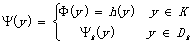Then Ψ|K = Φ|K. Therefore, the following claims show that ↓Ψ is as required.

Claim 4: For every y D, dH(↓Ψ(y), ↓Φ(y)) ≤ 4δ(y) < ε.

It can be proved similarly as the proof of [4, Proposition 1].

Claim 5: ↓Ψ: D →↓USC(Y) is a Z-embedding.

Since D is compact, it suffices to prove the following three aspects.

(1). ↓Ψ is continuous.

It follows from Claims 2, 3 and 4.

(2). Ψ is an injection.

For any y1, y2D with y1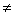y2, we shall show Ψ(y1)Ψ(y2). By the symmetry, we only consider the following three cases.

Case 1. y1, y2K. This fact is trivial.

Case 2. y1K and y2D\K. Then, by Claim 4,

dH(↓Ψ(y2),↓Φ(y2)) ≤ 4δ(y2).

On the other hand, it follows from the definition of δ that

dH(↓Φ(y1),↓Φ(y2)) ≥ dH(↓Φ(K),↓Φ(y2)) ≥ 5δ(y2) > 0.

We conclude that Ψ(y1) = Φ(y1)Ψ(y2).

Case 3. y1, y2D\K. If Ψ(y1) = Ψ(y2), then

δ(y1)=Ψ(y1)(x)=Ψ(y2)(x)=δ(y2)0. Thus there exists k such that y1, y2Dk and ϕk(y1) = ϕk(y2).

On the other hand, for every iN+,

Ψk(y1)(x2k+i) = Ψ(y1)(x2k+i)= Ψ(y2)(x2k+i) = Ψk(y2)(x2k+i).

Thus, if ϕk(y1)=ϕk(y2)=1, then α(y1)(m)=α(y2)(m) for every mN+ by δ(y1)=δ(y2) and the definition of Ψk(y1)(x2k+i) and Ψk(y2)(x2k+i) for every even number i with i≥8. If ϕ(y1) =ϕk(y2)1, then α(y1)(m)=α(y2)(m) for every mN+ by δ(y1)=δ(y2) and the definition of Ψk(y1)(x2k+i) and Ψk(y2)(x2k+i) for every even number i with i≥4. Thus α(y1) = α(y2) in both cases. Since α: D → Qu is injective, we have that y1=y2 which contradicts with the assumption of y1y2.

(3). ↓Ψ(D) is a Z-set of ↓USC(Y).

↓Ψ(D) is compact by (1). Noticing that, for every yDk, Ψ(y)(x′2k+4) = Ψk(y)(x′2k+4)=0, it follows from Lemma 6 that ↓Ψ(D) is a Z-set.

Claim 6: Ψ−1(USC(X))\K=B\K and Ψ−1(C(X))\K=A\K.

In fact, for every yD\K, there exists k such that yDk. It follows form the definition of Ψ and Claim 1. We are done.

4. Conclusion

For any bounded open interval X in the Euclidean space E1, ↓USC(X) is homeomorphic to s  and ↓C(X) is homeomorphic to c0 . However in this paper, it is proved that (↓USC(X), ↓C(X)) is pair homeomorphic to the pairwhich is not pair homeomorphic to the pair (s, c0).

Acknowledgements

This work is supported by National Natural Science Foundation of China Youth Science Foundation Project (No. 11505042).

References

1. R. D. Anderson, "Hilbert space is homeomorphic to countable infinite product of line," Bull. Amer. Math. Soc. vol.72, pp.515-519.
2. M. I. Kadec, "On topological equivalence of separable Banach spaces," Soviet Math. Dokl, vol. 7, pp. 319-322.
3. T. Dobrowolski, W. Marciszewski, J. Mogilski, On topopogical classification of function spaces Cp(X) of low Borel complexity, Trans. Amer. Math. Soc., vol. 678, pp. 307-324.
4. Z. Q. Yang, "The hyperspace of the regions below of continuous maps is homeomorphic to c0," Topology Appl., vol. 153, pp. 2908-2921.
5. Z. Q. Yang, and X.E. Zhou, "A pair of spaces of upper semi-continuous maps and continuous maps," Topology Appl. vol. 154, pp.1737-1747.
6. Z. Q. Yang and N. D. Wu, "The hyperspace of the regions below of continuous maps from S×S to I, Questions and Answers in General Topology," vol. 26, pp. 29-39.
7. Z. Q. Yang and N. D. Wu, "A topological position of the set of continuous maps in the set of upper semicontinuous maps," Sci. China, Ser. A, vol. 52, pp. 1815-1828.
8. Z. Yang, "The hyperspace of the regions below of all lattice-value continuous maps and its Hilbert cube compactification," Sci. China Ser. A, vol. 48, pp. 469-484.
9. Z. Q. Yang, and P. F. Yan, "Topological classification of function spaces with the Fell topology I," Topology Appl., vol. 178, pp. 146–159.
10. Z. Q. Yang, Y. M. Zheng, and J. Y. Chen, "Topological classification of function spaces with the Fell topology II," Topology Appl., vol. 187, pp. 82–96.
11. Z. Q. Yang, L. Z. Chen, and Y. M. Zheng, "Topological classification of function spaces with the Fell topology III," Topology Appl., vol. 197, pp. 112–132.
12. Y. J. Zhang and Z. Q. Yang, "Hyperspaces of the Regions Below of Upper Semi-continuous Maps on Non-compact Metric Spaces," Advances in Mathematics (China), vol. 39, pp. 352-360.
13. N. D. Wu, and Z. Q. Yang, "Spaces of Continuous Maps on a Class of Noncompact Metric Spaces," Advances in Mathematics (China), vol 42, pp. 535-541.
14. R. Cauty, and T. Dobrowolski, "Applying coordinate products to the topological identification of normed spaces," Trans. Amer. Math. Soc., vol. 337, pp. 625-649.
15. Van Mill J., The Infinite-Dimensional Topology of Function Spaces, Amsterdam: North-Holland Math. Library 64, Elsevier Sci. Publ. B. V., 2001.
16. Van Mill J., Infinite-Dimensional Topology, Prerequisites and Introduction, Amsterdam: North-Holland Math. Library 43, Elsevier Sci. Publ. B. V., 1989.
17. M. Bestvina, and J. Mogilski, "Characterizing certain incomplete infinite-dimensional absolute retracts," Michigan Math. J., vol. 33, pp. 291-313.
18. R. Cauty, T. Dobrowolski and W. Marciszewski, "A contribution to the topological classification of the spaces CP(X)". Fund. Math. vol. 142, pp. 269-301.
19. Z. Q. Yang, S.R.Hu, and G. Wei, "Topological structures of the space of continuous functions on a non-compact space with the Fell Topology," Topology proceedings, vol. 41, pp. 17-38.

 Contents 1. 2. 3. 3.1. 3.2. 4.
Article ToolsAbstractPDF(219K)